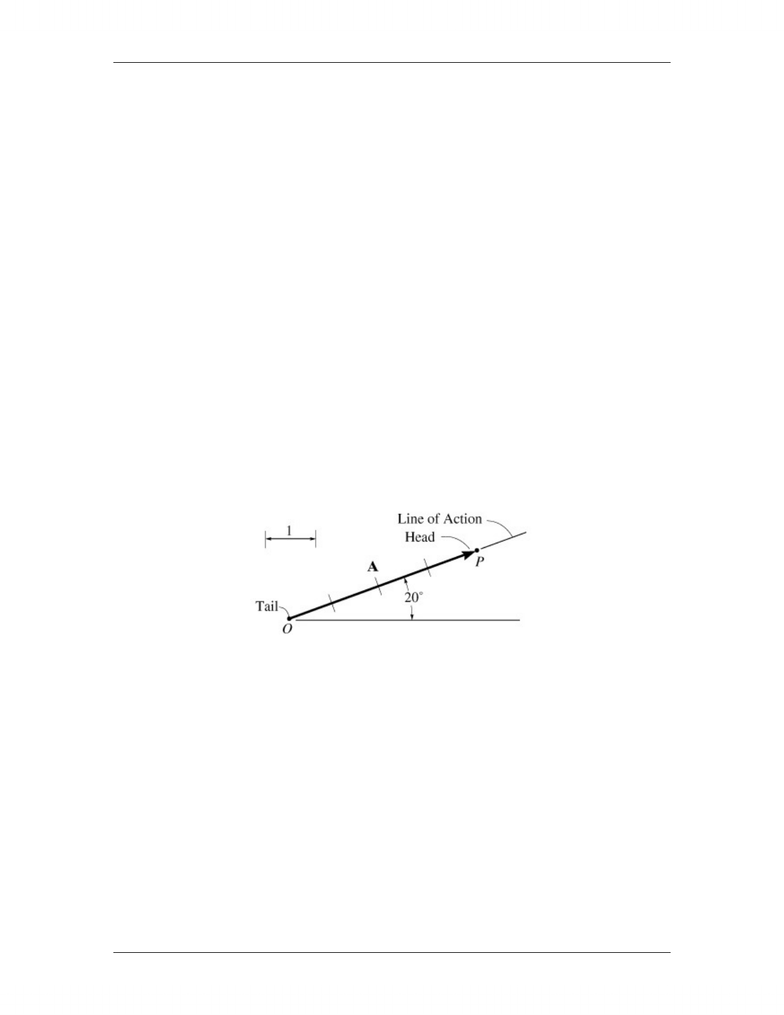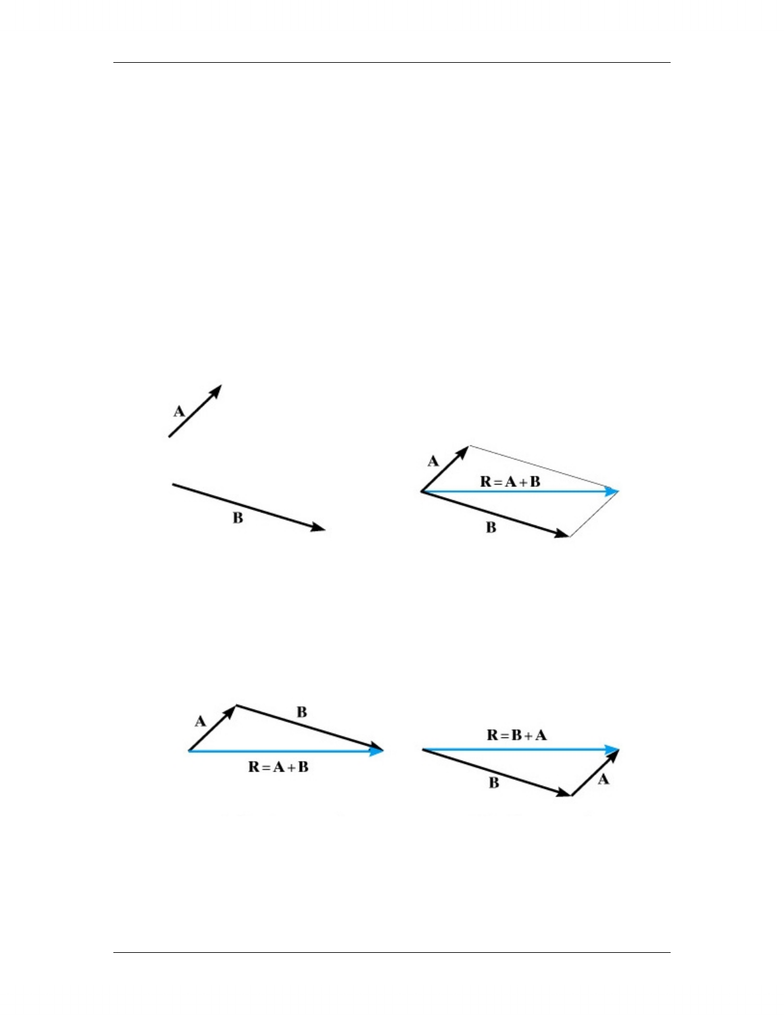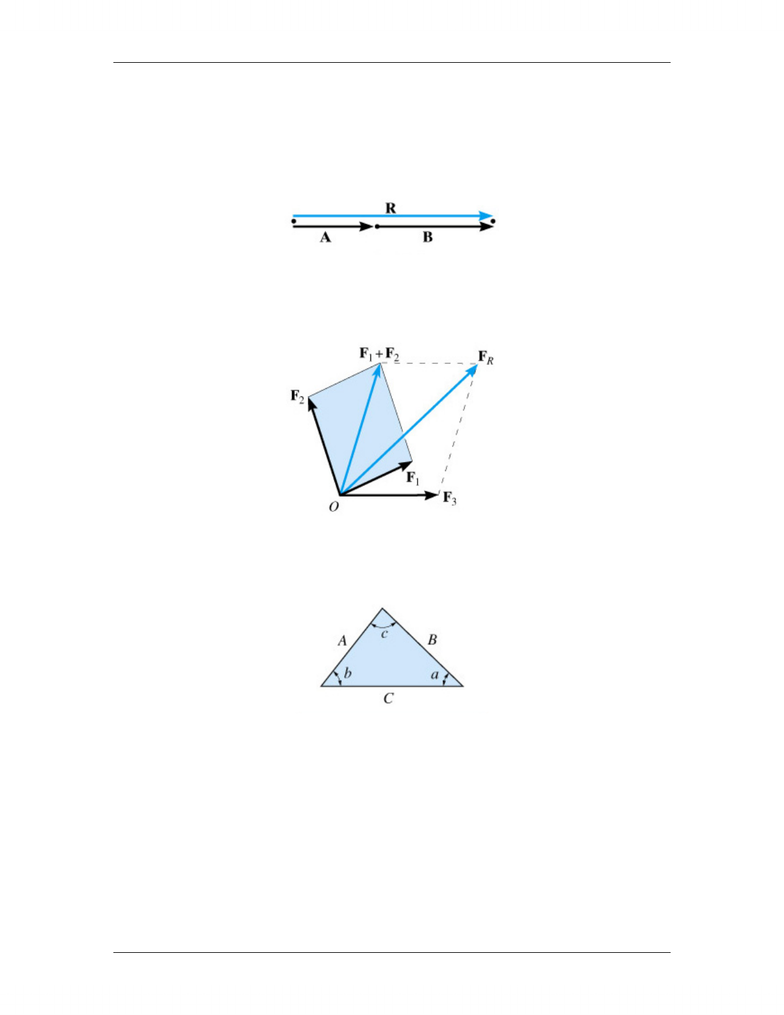# Engineering Science 1022A/B/Y Lecture Notes - Cartesian Coordinate System, Parallelogram Law, Unit Vector

71 views26 pages
School
Western University
Department
Engineering Science
Course
Engineering Science 1022A/B/Y
ProfessorES 1022y Engineering Statics Force Vectors
6
Force Vectors
In this course forces (acting along the vector axis), moments (rotation about the vector
axis), and position vectors (moving along the vector axis) are all vector quantities. A
force, moment or position vector has:
A vector quantity can be represented graphically by an arrow that shows its magnitude,
direction and sense.
Magnitude: characterized by size in some units, e.g. 34 N; represented by length of the
arrow according to some scale, say, 1 cm = 10 N 3.4 cm = 34 N.
Direction: the angle between a reference axis and the arrow's line of action.
Sense: indicated by the arrowhead (one of two possible directions)
A Word on Vector Notation
In the lecture notes and text book a vector quantity is indicated by a letter in boldface
type (F), while the magnitude of a vector is denoted by an italicized letter (F). For
handwritten work a vector is usually indicated by drawing an arrow above the letter
representing the vector, thus
Similarly, unit vectors can be denoted in handwritten work by drawing a hat symbol
above the letter to give
A magnitude, a direction, a sense, a point of
application
Unlock document

This preview shows pages 1-3 of the document.
Unlock all 26 pages and 3 million more documents.ES 1022y Engineering Statics Force Vectors
7
Consider two force vectors A and B. We want to add them together to find the vector
sum, or resultant force vector, R such that
We can do this using one of two methods.
Parallelogram law:
If the two forces A and B are represented by the adjacent sides of a parallelogram, then
the diagonal of the parallelogram is equal to the vector sum of the two forces.
Triangle of forces:
Special case of the parallelogram law.
R = A + B = B +
A
Unlock document

This preview shows pages 1-3 of the document.
Unlock all 26 pages and 3 million more documents.ES 1022y Engineering Statics Force Vectors
8
As a special case, if the two vectors A and B are collinear, that is
the parallelogram law reduces to an algebraic or scalar addition
Where we have three or more forces we can either use repeated applications of the
parallelogram law or a force polygon to find the resultant force.
We can also use trigonometry to add two force vectors together using the sine and cosine
laws. Consider a triangle with sides of length A, B, and C, and corresponding interior
angles a, b, and c.
Sine law:
Cosine law:
Both vectors have the same line of action
Unlock document

This preview shows pages 1-3 of the document.
Unlock all 26 pages and 3 million more documents.

## Document Summary

In this course forces (acting along the vector axis), moments (rotation about the vector axis), and position vectors (moving along the vector axis) are all vector quantities. A vector quantity can be represented graphically by an arrow that shows its magnitude, direction and sense. Magnitude: characterized by size in some units, e. g. 34 n; represented by length of the arrow according to some scale, say, 1 cm = 10 n 3. 4 cm = 34 n. Direction: the angle between a reference axis and the arrow"s line of action. Sense: indicated by the arrowhead (one of two possible directions) In the lecture notes and text book a vector quantity is indicated by a letter in boldface type (f), while the magnitude of a vector is denoted by an italicized letter (f). For handwritten work a vector is usually indicated by drawing an arrow above the letter representing the vector, thus.